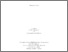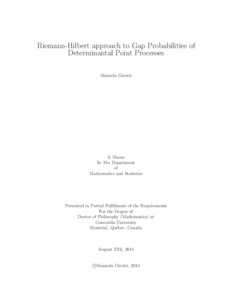Title:

# Riemann-Hilbert approach to Gap Probabilities of Determinantal Point Processes

Girotti, Manuela (2014) Riemann-Hilbert approach to Gap Probabilities of Determinantal Point Processes. PhD thesis, Concordia University.Preview
Text (application/pdf)
Girotti_PhD_F2014.pdf - Accepted Version
2MB

## Abstract

In this work, we study problems related to gap probabilities of certain universal determinantal point processes. The study of gap probabilities can be addressed in two directions: derivation of a Lax formulation of PDEs, as in the first two works presented here, and study of asymptotic behaviour, as in the last work. In order to achieve such results, the powerful theory of Riemann-Hilbert problem will be widely implemented.

We first consider the gap probability for the Bessel process in the single-time and multi-time case. We prove that the scalar and matrix Fredholm determinants of such process can be expressed in terms of determinants of integrable kernels in the sense of Its-Izergin-Korepin-Slavnov and thus related to suitable Riemann-Hilbert problems. In the single-time case, we construct a Lax pair formalism and we derive a Painleve' III equation related to the Fredholm determinant.

Next, we consider the problem of the gap probabilities for the Generalized Bessel process in the single-time and multi-time case, a determinantal process which arises as critical limiting kernel in the study of self-avoiding squared Bessel paths. As in the Bessel case, we connect the gap probability to a Riemann-Hilbert problem (derived from an IIKS kernel) on one side and to the isomonodromic tau-function on the other side.

In particular, in the single-time case we construct a Lax pair formalism and in the multi-time case we explicitly define a completely new multi-time kernel and we proceed with the study of gap probabilities as in the single-time case.

Finally, we investigate the gap probabilities of the single-time Tacnode process. Through steepest descent analysis of a suitable Riemann-Hilbert problem, we show that under appropriate scaling regimes the gap probability of the Tacnode process degenerates into a product of two independent gap probabilities of the Airy process.

Divisions: Concordia University > Faculty of Arts and Science > Mathematics and Statistics Thesis (PhD) Girotti, Manuela Concordia University Ph. D. Mathematics 27 August 2014 Bertola, Marco 978910 MANUELA GIROTTI 26 Nov 2014 14:25 18 Jan 2018 17:47
All items in Spectrum are protected by copyright, with all rights reserved. The use of items is governed by Spectrum's terms of access.

Repository Staff Only: item control pageResearch related to the current document (at the CORE website)
Back to top